# Lisp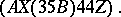Atoms are non-empty words over the alphabet of the language that do not contain brackets or gaps and denote variables, constants, functions, or themselves. Lists and atoms for which it makes sense to talk about their values are called expressions. The first element of a list — an expression— is an atom that denotes a function or a list that represents a function. The remaining elements of the listare expressions that are taken as arguments of the function, and the value ofis the result of taking a function from its arguments. The values of variables and constants are understood in the usual way. The representation of a function has the form of a list, where lambda is a fixed atom,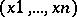is a list of bound variables that denote the arguments of a function, and exp is the expression that computes the value of a function and contains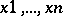as free variables.
Among the fundamental functions of Lisp are: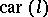and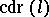, which point out the first element of the listand its "remainder" ;, which points outas its value;, which "extends" the listby the list;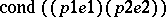, which chooses as its value the value of the expression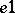if the predicate expression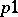is true, takes that of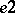if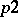is true and takes that ofif bothandare false. The fundamental predicates are:, which verifies whetheris an atom;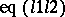, which is true ifand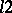are equal atoms; and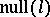, which is true ifturns out to be the empty list. The fundamental functions and predicates form a collection of tools that is sufficient to realize other constructions of algorithmic languages in Lisp, for example, the construction of ascribing a variable value to an expression or relating with an atom the representation of the function denoted by it. A program in Lisp is an arbitrary list formed by expressions.